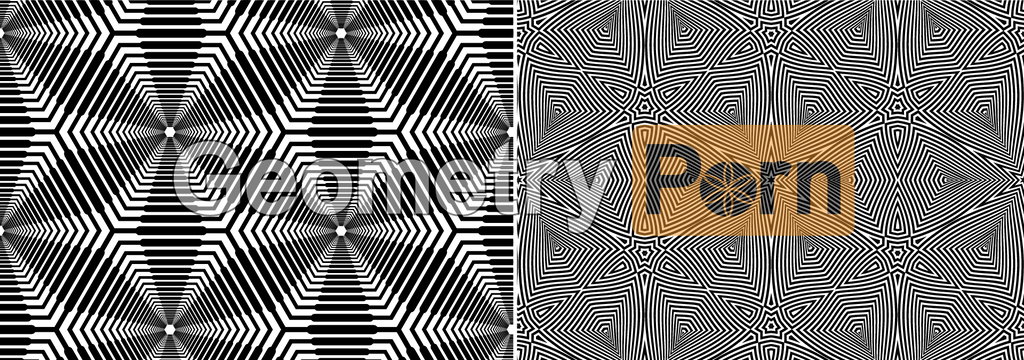# FRACTAL GEOMETRYA fractal is a non-regular geometric shape that has the same degree of non-regularity on all scales. Fractals can be thought of as never-ending patterns. The term "fractal" was coined by Benoit Mandelbrot in 1975. It comes from the Latin fractus, meaning an irregular surface like that of a broken stone. Fractals are the kind of shapes we see in nature.

We can describe a right triangle by the Pythagorean theorem, but finding a right triangle in nature is a different matter altogether. We find trees, mountains, rocks and cloud formations in nature, but what is the geometrical formula for a cloud? Fractal geometry, chaos theory and complex mathematics attempt to answer questions like these. Science continues to discover an amazingly consistent order behind the universe's most seemingly chaotic phenomena.

The word "fractal" often has different connotations for the lay public as opposed to mathematicians, where the public are more likely to be familiar with fractal art than the mathematical concept. The mathematical concept is difficult to define formally, even for mathematicians, but key features can be understood with little mathematical background.

Mathematicians have attempted to describe fractal shapes for over one hundred years, but with the processing power and imaging abilities of modern computers, fractals have enjoyed a new popularity because they can be digitally rendered and explored in all of their fascinating beauty. Fractals are being used in schools as a visual aid to teaching math, and also in our popular culture as computer-generated surfaces for landscapes and planetary surfaces in the movie industry. The use of algorithms to generate fractals can produce complex visual patterns for computer generated imagery (CGI) applications.